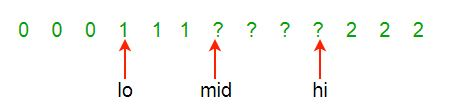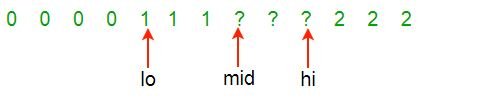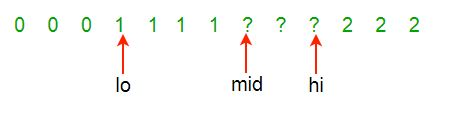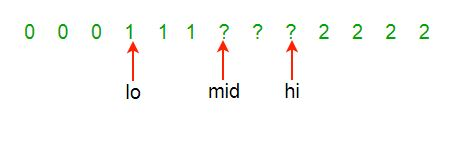# Javascript Program For Sorting An Array Of 0s, 1s and 2s

• Last Updated : 16 Dec, 2021

Given an array A[] consisting 0s, 1s and 2s. The task is to write a function that sorts the given array. The functions should put all 0s first, then all 1s and all 2s in last.
Examples:

```Input: {0, 1, 2, 0, 1, 2}
Output: {0, 0, 1, 1, 2, 2}

Input: {0, 1, 1, 0, 1, 2, 1, 2, 0, 0, 0, 1}
Output: {0, 0, 0, 0, 0, 1, 1, 1, 1, 1, 2, 2}```

A simple solution is discussed in this(Sort an array of 0s, 1s and 2s (Simple Counting)) post.
Method 1:

Approach:The problem is similar to our old post Segregate 0s and 1s in an array, and both of these problems are variation of famous Dutch national flag problem.
The problem was posed with three colours, here `0′, `1′ and `2′. The array is divided into four sections:

1. a[1..Lo-1] zeroes (red)
2. a[Lo..Mid-1] ones (white)
3. a[Mid..Hi] unknown
4. a[Hi+1..N] twos (blue)
5. If the ith element is 0 then swap the element to the low range, thus shrinking the unknown range.
6. Similarly, if the element is 1 then keep it as it is but shrink the unknown range.
7. If the element is 2 then swap it with an element in high range.

Algorithm:

1. Keep three indices low = 1, mid = 1 and high = N and there are four ranges, 1 to low (the range containing 0), low to mid (the range containing 1), mid to high (the range containing unknown elements) and high to N (the range containing 2).
2. Traverse the array from start to end and mid is less than high. (Loop counter is i)
3. If the element is 0 then swap the element with the element at index low and update low = low + 1 and mid = mid + 1
4. If the element is 1 then update mid = mid + 1
5. If the element is 2 then swap the element with the element at index high and update high = high – 1 and update i = i – 1. As the swapped element is not processed
6. Print the output array.

Dry Run:
Part way through the process, some red, white and blue elements are known and are in the “right” place. The section of unknown elements, a[Mid..Hi], is shrunk by examining a[Mid]:Examine a[Mid]. There are three possibilities:
a[Mid] is (0) red, (1) white or (2) blue.
Case (0) a[Mid] is red, swap a[Lo] and a[Mid]; Lo++; Mid++Case (1) a[Mid] is white, Mid++Case (2) a[Mid] is blue, swap a[Mid] and a[Hi]; Hi–Continue until Mid>Hi.

Implementation:

## Javascript

 ``

Output:

```array after segregation
0 0 0 0 0 1 1 1 1 1 2 2 ```

Complexity Analysis:

• Time Complexity: O(n).
Only one traversal of the array is needed.
• Space Complexity: O(1).
No extra space is required.

Method 2:

Approach: Count the number of 0s, 1s and 2s in the given array. Then store all the 0s in the beginning followed by all the 1s then all the 2s.

Algorithm:

1. Keep three counter c0 to count 0s, c1 to count 1s and c2 to count 2s
2. Traverse through the array and increase the count of c0 if the element is 0,increase the count of c1 if the element is 1 and increase the count of c2 if the element is 2
3. Now again traverse the array and replace first c0 elements with 0, next c1 elements with 1 and next c2 elements with 2.

Implementation:

## Javascript

 ``

Output:

`0 0 0 0 0 1 1 1 1 1 2 2`

Complexity Analysis:

• Time Complexity: O(n).
Only two traversals of the array is needed.
• Space Complexity: O(1).
As no extra space is required.

Please refer complete article on Sort an array of 0s, 1s and 2s for more details!

My Personal Notes arrow_drop_up